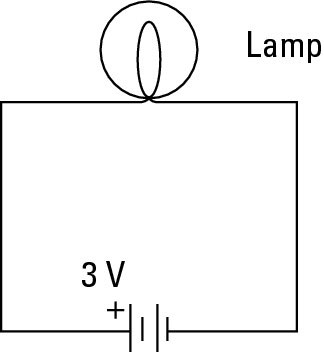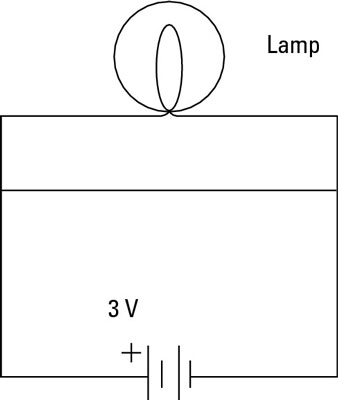##### Circuit Analysis For DummiesAn electronic circuit is a complete course of conductors through which current can travel. Circuits provide a path for current to flow. To be a circuit, this path must start and end at the same point. In other words, a circuit must form a loop. An electronic circuit and an electrical circuit has the same definition, but electronic circuits tend to be low voltage circuits.

For example, a simple circuit may include two components: a battery and a lamp. The circuit allows current to flow from the battery to the lamp, through the lamp, then back to the battery. Thus, the circuit forms a complete loop.Of course, circuits can be more complex. However, all circuits can be distilled down to three basic elements:

• Voltage source: A voltage source causes current to flow like a battery, for instance.

• Load: The load consumes power; it represents the actual work done by the circuit. Without the load, there's not much point in having a circuit.

The load can be as simple as a single light bulb. In complex circuits, the load is a combination of components, such as resistors, capacitors, transistors, and so on.

• Conductive path: The conductive path provides a route through which current flows. This route begins at the voltage source, travels through the load, and then returns to the voltage source. This path must form a loop from the negative side of the voltage source to the positive side of the voltage source.

The following paragraphs describe a few additional interesting points to keep in mind as you ponder the nature of basic circuits:

• When a circuit is complete and forms a loop that allows current to flow, the circuit is called a closed circuit. If any part of the circuit is disconnected or disrupted so that a loop is not formed, current cannot flow. In that case, the circuit is called an open circuit.

Open circuit is an oxymoron. After all, the components must form a complete path to be considered a circuit. If the path is open, it isn't a circuit. Therefore, open circuit is most often used to describe a circuit that has become broken, either on purpose (by the use of a switch) or by some error, such as a loose connection or a damaged component.

• Short circuit refers to a circuit that does not have a load. For example, if the lamp is connected to the circuit but a direct connection is present between the battery's negative terminal and its positive terminal, too.Current in a short circuit can flow at dangerously high levels. Short circuits can damage electronic components, cause a battery to explode, or maybe start a fire.

The short circuit illustrates an important point about electrical circuits: it is possible — common, even — for a circuit to have multiple pathways for current to flow. The current can flow through the lamp as well as through the path that connects the two battery terminals directly.

Current flows everywhere it can. If your circuit has two pathways through which current can flow, the current doesn't choose one over the other; it chooses both. However, not all paths are equal, so current doesn't flow equally through all paths.

For example, current will flow much more easily through the short circuit than it will through the lamp. Thus, the lamp will not glow because nearly all of the current will bypass the lamp in favor of the easier route through the short circuit. Even so, a small amount of current will flow through the lamp.